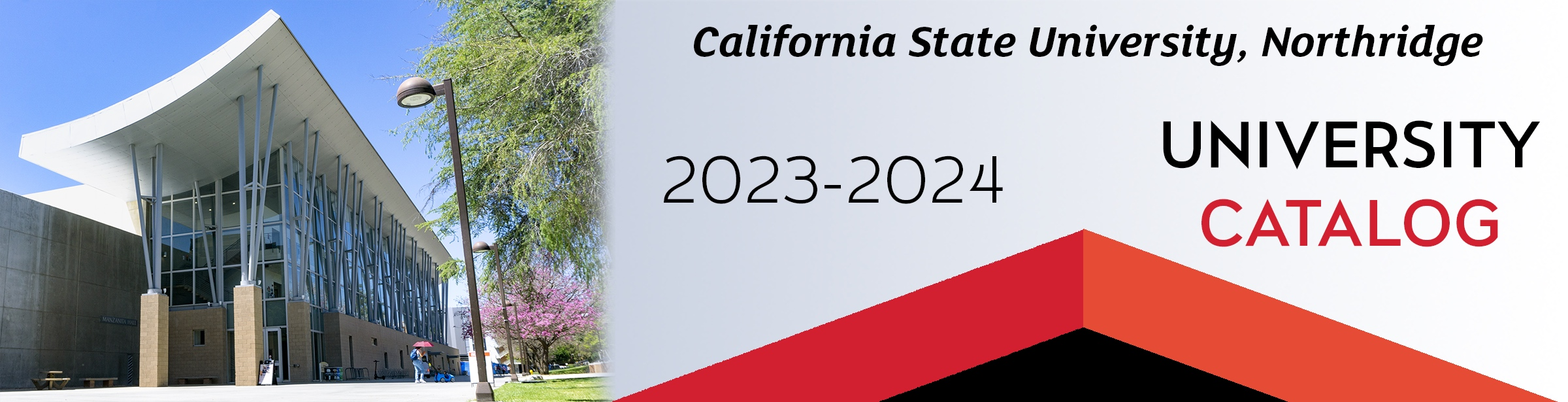## Course: ME 280. Differential Equations for Mechanical Engineers (3)

Prerequisite: MATH 150B. Recommended Preparatory Course: MATH 250. Introduction to differential equations used in engineering applications. Engineering analysis of physical systems described by differential equations: pendulums, mass-spring damper, R-L-C circuits, vibrations, beam bending, heat transfer, and hydrodynamics. Exploration of solution techniques, including undetermined coefficients, power series, and Laplace Transform. Determination of initial/boundary conditions. Linear algebra and solution of systems of differential equations. Introduction to partial differential equations and separation of variables. Not available to students with credit for MATH 280 or ECE 280.

### ME 280

Class NumberLocationDayTime